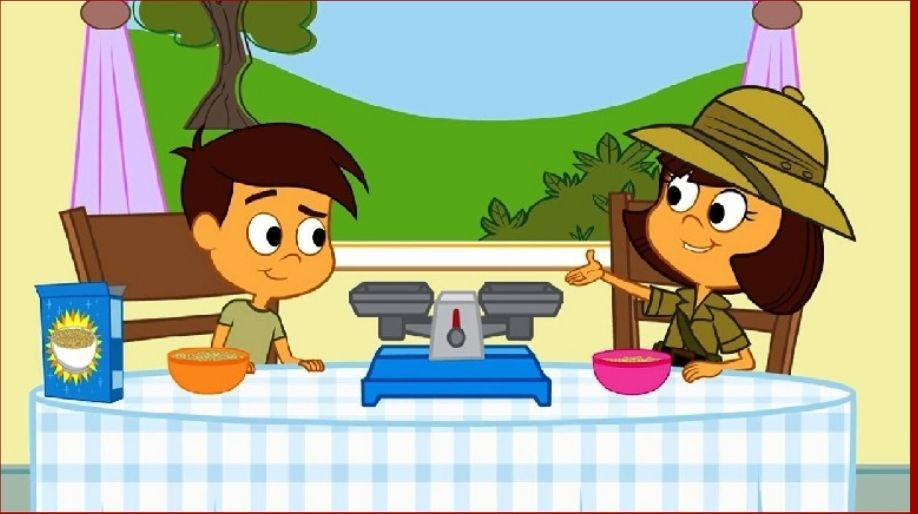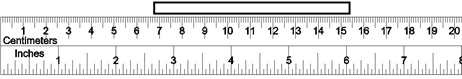Register for Maths, Science, English, Reasoning Olympiad Exams Register here | Book Free Demo for Live Olympiad Classes here | Check Olympiad Exam Dates here | Buy Practice Papers for IMO, IOM, HEO, IOEL etc here | Login here to participate in all India free mock test on every Saturday

# Measurement - Length, Mass, Capacity, Volume• Length: Metric unit of lengths are used in meters, kilometers, centimeters, millimeters, etc.

 10 millimeters (mm) 1 centimeter (cm) 10 centimeters 100 millimeters 100 centimeters 1 meter (m) 100 meters 1 kilometer (km) 1 Ft 12 Inch 1 Mile 5280 Foot

• Capacity: It can be measured in liters, kiloliters, etc.

 Kiloliter (kl) 1,000 liters Liter (l) 1 liter Centiliter (cl) 0.01 liter Milliliter (ml) 0.001 liter 1 Pound(lb) 16 Ounce (oz)

• Mass: Mass is generally the measurement of weight and there are three main units of mass considered as M, Kg, S.

 M The meter of length Kg The kilogram of mass S The second for timed

Example:

• Convert 7.5 km into meters.

Sol: 1 km = 1000 m

7.5 km = (7.5×1000) m = 7500 m

• Convert 753 mm into cm

Sol: 1 mm = 0.1 cm = 1/10 cm

753 mm = 75.3 cm

• Convert 746 kl into liters.

Sol: 1 liter = 1000 kl

746 kl = 746/1000 liters = 0.746 liters

• How many foot are there is 48 inches?

Sol: 1 Foot = 12 inches

48 inches = (48/12) foot = 4 foot

• Convert 9.7 kg into grams.

Sol: 1 kg = 1000 gm.

IMPORTANT: Units should be same while addition and subtraction of numbers.

9.7 kg = 9.7×1000 = 9700 grams

Practice Exercise

• Seema runs 2.86 km every day. How far does Seema run in a week?

• Varun is 3.12 m tall. His younger brother is 28 cm taller than him. What is his brother’s height?

• The length of tower A is 335.6 m and the length of tower B is 456.4 m. By how many meters tower B is taller than tower A?

• Convert the following:
1. 02 m = ____ cm
2. 5436 m = ____ km
• 765 mm = ____ cm
1. 89 liter = ____ ml
2. 167 oz = ____ lb

1. 68 km 20 m and 23 km 21 m
2. 245 grams and 4.5 kg

• Subtract:
1. 750 grams from 8 kg
2. 238 liters from 6 kl

RECAP

• 1 km = 1000 m, 1 kg = 1000 gm, 1 kl = 1000 l
• 1 meter = 100 cm
• 1 cm = 10 mm
• 1 foot = 12 inches
• 1 Pound = 16 ounce
• Make sure the units of amounts should be same at the time of addition and subtraction. You cannot add 100 gm and 100 kg.

## Quiz for Measurement - Length, Mass, Capacity, Volume

 Q.1 Measure the length of the object in centimeter scale.a) 11 cm. b) 13 cm. c) 12 cm. d) 14 cm.
 Q.2 Measure the length of the object in centimeter scale.a) 8.5 cm. b) 8.9 cm. c) 9.1 cm. d) 8.7 cm.
 Q.3 Convert into millimeter: 2.47 cm. a) 34.7 mm. b) 24.7 mm. c) 22.7 mm. d) 26.7 mm.
 Q.4 Convert into meter: 999 mm. a) 0.99 m. b) 0.999 m. c) 9.99 m. d) 99.9 m.
 Q.5 Convert into centimeter: 12 m. a) 1200 cm. b) 120 cm. c) 12000 cm. d) 0.12 cm.
 Q.6 Convert into kilometer: 19 mm. a) 0.0019 km. b) 0.019 km. c) 0.000019 km. d) 0.00019 km.
 Q.7 1.24 km.+ 89 cm. = __?__ km. a) 124.089 km. b) 1.2489 km. c) 1.24089 km. d) 12.489 km.
 Q.8 __?__ km. + 4.896 km. = 12.2 km. a) 7.034 km. b) 7.403 km. c) 7.34 km. d) 7.304 km.
 Q.9 145.2 m. x 11 = __?__ m. a) 1587.4 m. b) 1597.8 m. c) 1597.2 m. d) 1597.6 m.
 Q.10 7.70 km. ÷ 14 = __?__ m. a) 540 m. b) 530 m. c) 510 m. d) 550 m.